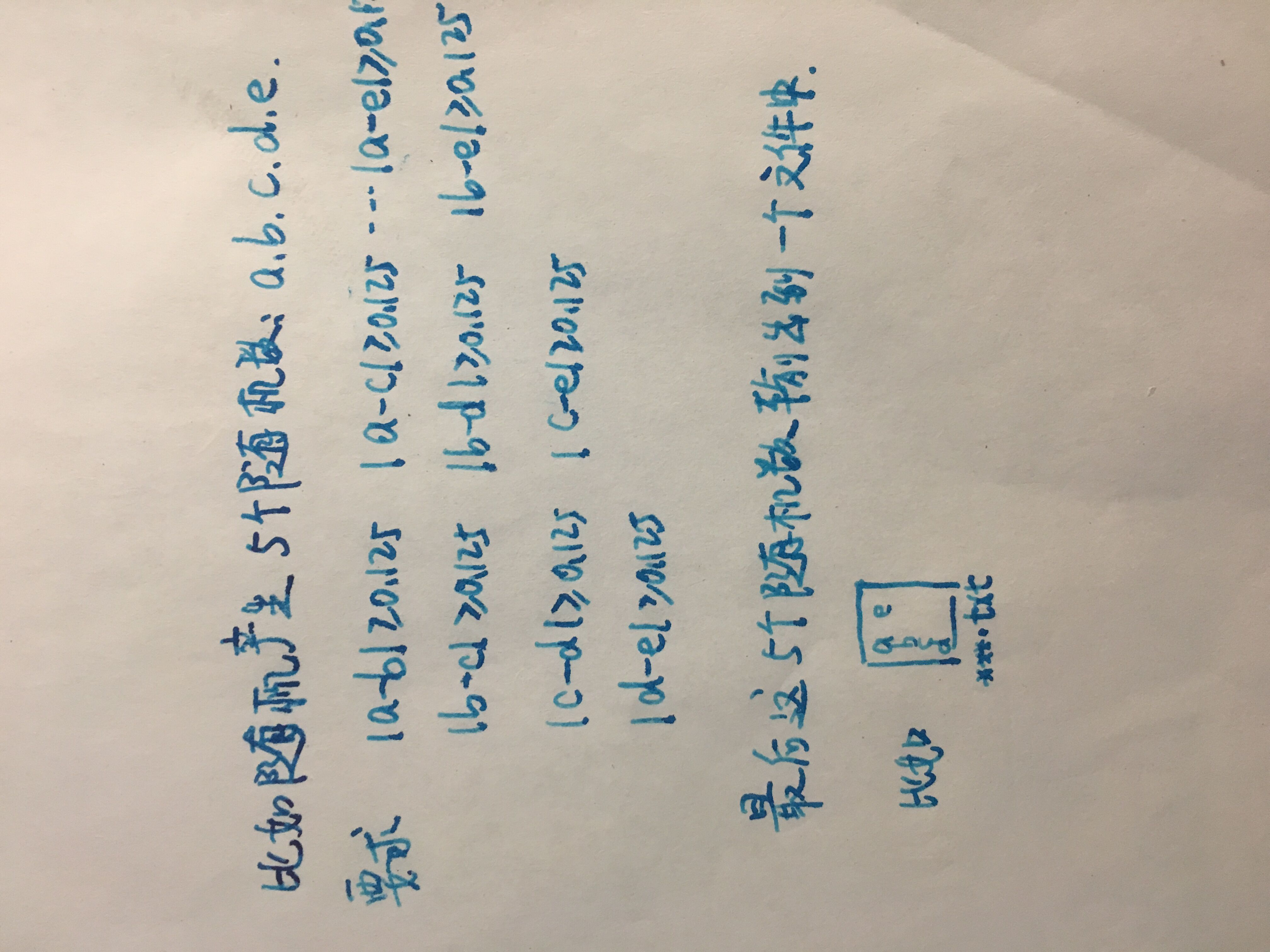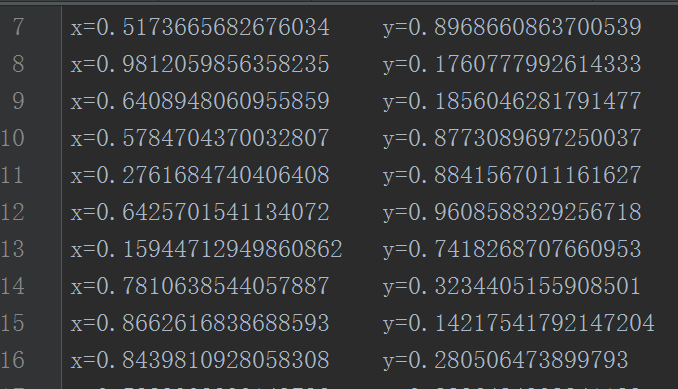python产生100个随机数,每个随机数之间的差值为固定值?4个回答2 年多之前 回复

import random

f = open("data_100.txt","a+",encoding="utf-8")

for x in range(100):
a = random.random()
b = random.random()
if a - b >=0.125 or b - a >=0.125:
f.write(str(a))
f.write(' ')
f.write(str(b))
f.write('\n')

f.close()

``````#!usr/bin/env python
#_*_ coding:utf-8 _*_

import random

with open('ana2.txt','a+') as f:
for i in range(100):
x=random.random()
y=random.random()
if abs(x-y)>=0.25:
f.write('\n'+'x='+str(x)+'\t'+'y='+str(y))

``````-

-